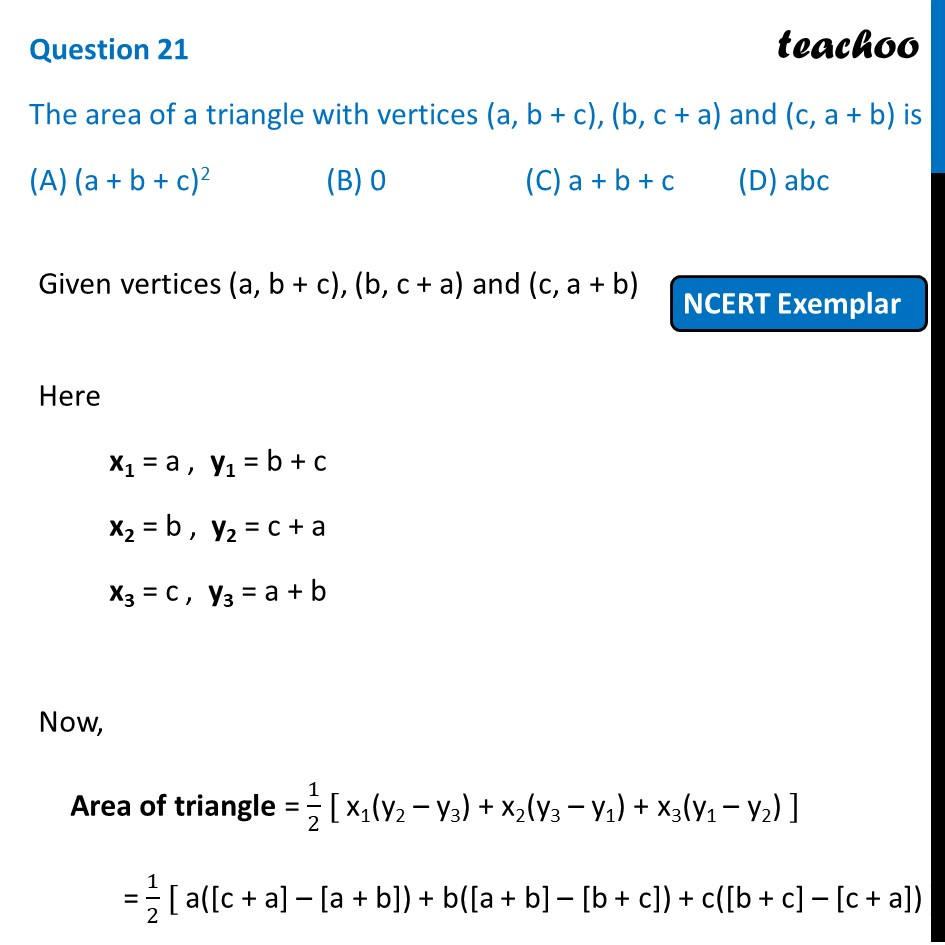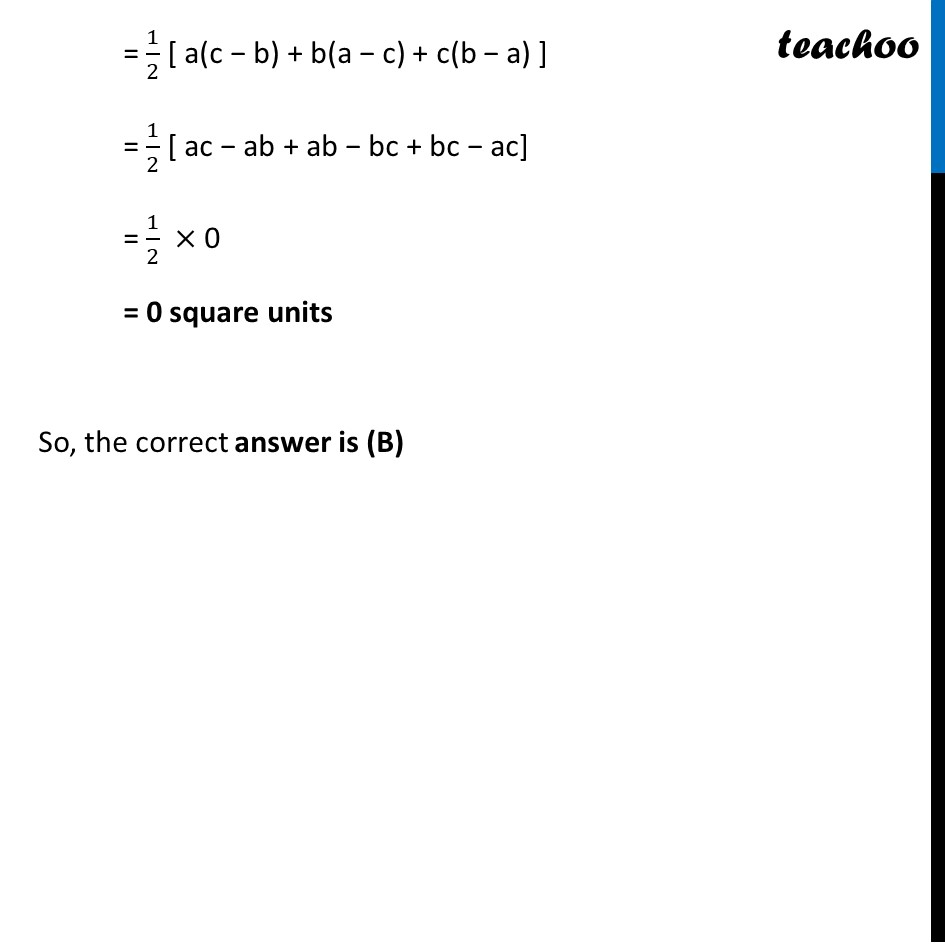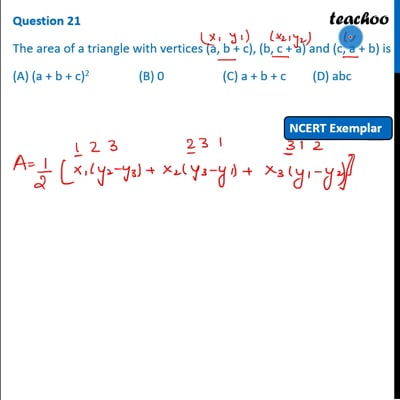NCERT Exemplar - MCQ

Chapter 7 Class 10 Coordinate Geometry
Serial order wise

## (D) abcThis video is only available for Teachoo black users

Learn in your speed, with individual attention - Teachoo Maths 1-on-1 Class

### Transcript

Question 21 The area of a triangle with vertices (a, b + c), (b, c + a) and (c, a + b) is (A) (a + b + c)2 (B) 0 (C) a + b + c (D) abc Given vertices (a, b + c), (b, c + a) and (c, a + b) Here x1 = a , y1 = b + c x2 = b , y2 = c + a x3 = c , y3 = a + b Now, Area of triangle = 1/2 [ x1(y2 – y3) + x2(y3 – y1) + x3(y1 – y2) ] = 1/2 [ a([c + a] – [a + b]) + b([a + b] – [b + c]) + c([b + c] – [c + a]) ] = 1/2 [ a(c − b) + b(a − c) + c(b − a) ] = 1/2 [ ac − ab + ab − bc + bc − ac] = 1/2 × 0 = 0 square units So, the correct answer is (B)